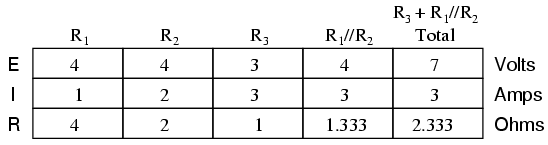# THEVENIN’S SUPERPOSITION THEOREM

May 3 • General • 5591 Views • 1 Comment on THEVENIN’S SUPERPOSITION THEOREM

Thevenin’s Superposition Theorem

What is thevenin’s superposition theorem??

Thevenin’s Superposition  Theorem is  useful in analyzing power  circuits where one particular resistor in the circuit (called the “load” resistor) is subject to change, and re-calculation of the circuit is necessary with each trial value of load resistance, to determine voltage across it and current through it. The concept  used in thevenin’s Superposition Theorem is to eliminate all, but one source of power within a network, using series/parallel analysis to determine voltage drops  within the modified network for each power source separately.For AC systems, the theorem can be applied to reactive impedences  as well as resistances. Then according to  thevenin’s Superposition Theorem once voltage drops and/or currents have been determined for each power source working separately, the values are all “superimposed” on top of each other (added algebraically) to find the actual voltage drops/currents with all sources active.

The Thévenin-equivalent voltage is the voltage at the output terminals of the original circuit. When calculating a Thévenin-equivalent voltage, the voltage divider principle is often useful, by declaring one terminal to be Vout. and the other terminal to be at the ground point.

The Thévenin-equivalent resistance is the resistance measured across points A and B “looking back” into the circuit. It is important to first replace all voltage- and current-sources with their internal resistances. For an ideal voltage source, this means replace the voltage source with a short circuit. For an ideal current source, this means replace the current source with an open circuit. Resistance can then be calculated across the terminals using the formulae for series and parallel . This method is valid only for circuits with independent sources. If there are dependent sources in the circuit, another method must be used such as connecting a test source across A and B and calculating the voltage across or current through the test source.

Steps to follow for Thevenin’s Theorem:

•  Remove the load resistor from the original circuit and calculate the voltage across the open connection points where the load resistor used to be.This is Thevenin’s voltage.
•  Find the Thevenin resistance by removing all power sources in the original circuit that is short circuiting the voltage sources and open circuiting the current sourses and calculate the total resistance between the open connection points.
•  Draw the Thevenin equivalent circuit, with the Thevenin voltage source in series with the Thevenin resistance.

Helps to know the output voltage  without analyzing the circuit again and again.

1. 1.      Initial Circuit
2. 2.      The circuit with only the 28 volt battery in effect. . .Analyzing the circuit with only the 28 volt battery, we obtain the following values for voltage and current:Analyzing the circuit with only the 7 volt battery, we obtain above  set of values for voltage and current.After applying thevenin’s superposition theorem:`Q1) Can Superposition theorem be applied to AC Circuits?`
`A1) Yes,  superposition  theorem can be applied to ac as well as dc circuits .`
`Q2)  Give the Application of Superposition theorem.`

A2) Helps to know the output voltage  without analyzing the circuit again and again.

Q3) Which of the following is non-linear circuit parameter ?\

a)      Resistor 2) capacitor 3) inductor 4) Diode

A3) Diode

### One Response to THEVENIN’S SUPERPOSITION THEOREM

1. Soubarna Biswas says:

Thevenin’s theorem is a very important theorem in case of the electrical circuits. One should have ample knowledge about this theorem who is of Electrical Department. This article has explained the theorem so nicely that if one reads this, the person will have no doubts about it.